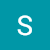# DSA-Recursion

`if (test for Base Case-I)   return Base Case Valueelsif (test for Base Case-II)   return Base Case Value IIDo something if neededMake recursive callDo Something if Need`

Recursion Problems:

Sorting:
1. Merge Sort:

2. Quick Sort:

Binary Search:

1. Inorder

2. Preorder

3. PostOrder

## Graph Traversals

2. Depth First Search

Tower of Hanoi:

--

--

# DSA-Data Structure

It is a particular way to store and organize data in a computer so that it can be used efficiently. The data structure can be classified into two ways:

1. Linear data structure
2. Non-linear data structure

## Abstract Data Types

User defined data structures combined with their operations are called Abstract Data types. It consists of two parts:
1. Declaration of data
2. Declaration of operations

## Algorithm

The two criteria for algorithms:
1. Correctness — Does the algorithm give solution in finite number of steps?
2. Efficiency — Resources needed in terms of memory and time.
Comparison of Algorithm: Rate of growth

Big — O notation: Upper Bound running time
Big — Ω notation: Lower Bound running time
(No practical importance)
Big — Θ notation: Average running time

--

--

# Codeforces 1389/A

## INSPECTION:

`Find two integers x and y such that l≤x<y≤r and l≤LCM(x,y)≤r.let x=lLCM(x,x*2) if x*2<=r is the smallest LCM(x,y).Trusting greedy,  x,y=l,l*2`

## CODE

`def solve(arr):    [x,y]=arr    if x*2>y:        print(-1,-1)    else:        print(x,x*2)t= int(input())for _ in range(t):    arr= list(map(int, input().rstrip().split()))    solve(arr)`

--

--

# Codeforces 1337/A

## Inspection:

1. High constraints. Looping (O (n³)) is not efficient.
`The next t lines describe test cases. Each test case is given as four space-separated integers a, b, c, d (1≤a≤b≤c≤d≤10^9).`

2. Rule of Triangle sides

`X+Y>Z `

3. When they satisfy the rule of Triangles

`Min X = a , Max X = bMin Y = b , Max Y = cMin(X+Y) = a+b , Max (X+Y) = b+c if there exists z such that c<= z<=d and z< Max(X+Y)or c<=z<=Min(d,b+c-1)=> Triangle exists. All z in this range is a valid one.`

4. If a triangle exists, find the sides .

`Assume z= Min(d,b+c-1)x,y=b,c if z==d and d<b+c-1:    z<x+y is Trueelse:    z<x+y is True too`

## CODE

`def solve(arr):    [a,b,c,d]=arr    z=min(d,b+c-1)    print(b,c,z)t= int(input())for _ in range(t):    arr= list(map(int, input().rstrip().split()))    solve(arr)`

--

--

# Leetcode 204. Count Primes (Number Theory)

INSPECTION

1. Nothing special in this problem. Sieve of Eratosthenes implementation.

SOLUTION

`import mathclass Solution:    def countPrimes(self, n: int) -> int:                if n<=1:            return 0                boolean_counter=[True for j in range(n+1)]        boolean_counter,boolean_counter=False,False        for i in range(2,int(math.sqrt(n))+1):            if (boolean_counter[i]==True and i*i<=n):                for j in range(i*i,n+1,i):                    boolean_counter[j]=False        prime_count=0        for i in range(2,n):            if boolean_counter[i]:                prime_count+=1        return prime_count`

--

--